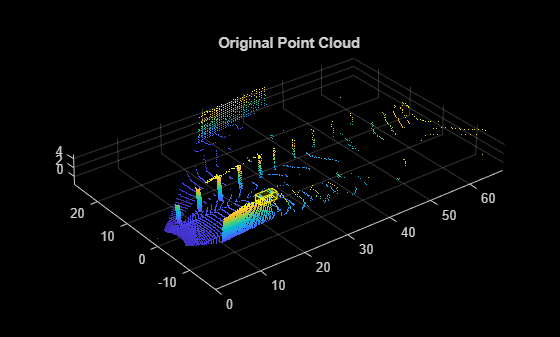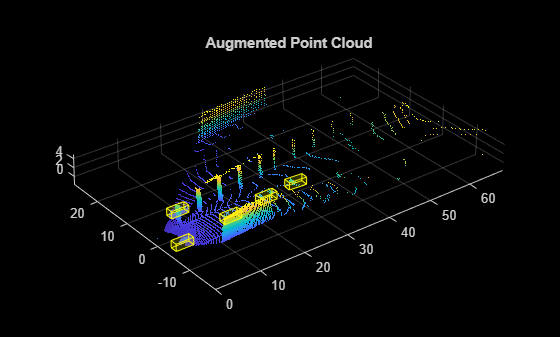# pcBboxOversample

Randomly augment point cloud data using objects

## Syntax

``augmentedPcBoxLabels = pcBboxOversample(pcBoxLabels,sampleData,classNames,totalObjects)``

## Description

example

````augmentedPcBoxLabels = pcBboxOversample(pcBoxLabels,sampleData,classNames,totalObjects)` randomly augments the specified point cloud data `pcBoxLabels` using objects with specified class names `classNames` from the training data datastore `sampleData`.```

## Examples

collapse all

Load a point cloud and its class labels into the workspace.

```unzip("sampleWPIPointClouds.zip",tempdir); dataLocation = fullfile(tempdir,"sampleWPIPointClouds"); load("sampleWPILabels.mat","trainLabels");```

Create a datastore for training data.

```pcds = fileDatastore(dataLocation,"ReadFcn",@(x) pcread(x)); blds = boxLabelDatastore(trainLabels); trainingData = combine(pcds,blds);```

Define the class names to sample from the input data. Use the `sampleLidarData` function to sample the corresponding bounding boxes.

```classNames = {'car'}; [pcdsSampled,bldsSampled] = sampleLidarData(trainingData,classNames,Verbose=false); cdsSampled = combine(pcdsSampled,bldsSampled);```

Read a point cloud from the training data.

```pcBoxLabels = read(trainingData); figure pcshow(pcBoxLabels{1,1}.Location) showShape(cuboid=pcBoxLabels{1,2},Opacity=0.1, ... Color="yellow",LineWidth=0.5); title("Original Point Cloud")```Augment the point cloud data `pcBoxLabels` with points sampled from the datastore `cdsSampled` by using the `pcBboxOversample` function.

```totalObjects = 5; augmentedPcBoxLabels = pcBboxOversample(pcBoxLabels,cdsSampled,classNames,totalObjects); figure pcshow(augmentedPcBoxLabels{1,1}.Location) showShape(cuboid=augmentedPcBoxLabels{1,2},Opacity=0.1, ... Color="yellow",LineWidth=0.5); title("Augmented Point Cloud")```## Input Arguments

collapse all

Input point cloud data, specified as a 1-by-3 cell array. The cells contain the point cloud, the bounding box annotations and the bounding box categories, respectively.

Training data, specified as a valid datastore object or table.

• If you specify a datastore object, your data must be set up such that using the `read` function on the datastore object returns a cell array or table with three columns. Each row corresponds to an object, and the columns must follow this format.

• First column — Sampled points, specified as a cell array.

• Second column — Bounding box, specified as a cell array containing a 9-element vector of the form [x y z length width height roll pitch yaw], representing the location and dimensions of the bounding box for the sampled points.

• Third column — Label, specified as a cell array containing a categorical vector with the object class name.

You can use the `combine` function to combine two or more datastores. For more information on creating datastore objects, see the `datastore` function.

• If you use a table, the table must have two or more columns. The first column must contain point cloud file names with the file location. The point cloud files can be in any format supported by `pcread` function. Each of the remaining columns represent a single object class such as Car, or Truck containing a 9-element vector of the form [x y z length width height roll pitch yaw], specifying the location and dimensions of the bounding box corresponding to the sampled points in the point cloud.

Names of object classes, specified as a M-element vector of strings, M-element categorical vector, M-element cell array of character vectors. M is the number of object classes specified.

Total number of objects in each class of the output point cloud, specified as a positive scalar or M-element vector. When this value is a scalar, the function uses the same value for all classes. When you specify an M-element vector, each element specifies the number of objects of the corresponding class in the `classNames` argument.

## Output Arguments

collapse all

Augmented point cloud data, returned as a 1-by-3 cell array. The cells contain the augmented point cloud, the bounding box annotations, and the box categories, respectively.

## Algorithms

Lidar object detection techniques directly predict 3-D bounding boxes around objects of interest. Data augmentation helps you improve prediction accuracy and avoid overfitting issues while training.

You can perform ground truth data augmentation on point clouds using these steps.

1. Sample 3-D bounding boxes and the corresponding points from input training data using the `sampleLidarData` function.

2. Augment a point cloud randomly with the sampled bounding boxes by using the `pcBboxOversample` function. The function performs a collision test on the sampled boxes and the ground truth boxes of the input point cloud to avoid overlap.

This technique alleviates the class imbalance problem in lidar object detection.

## Version History

Introduced in R2022a